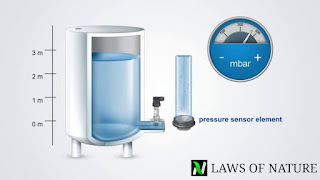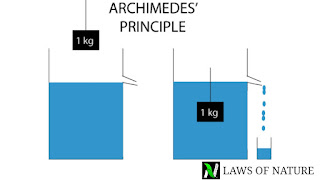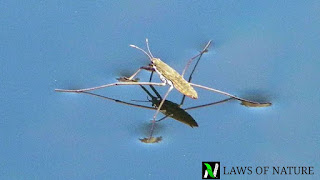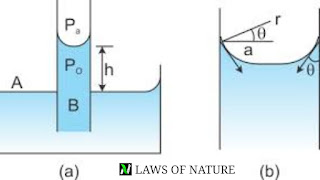# Hydrostatic : fluids at rest | concept booster , chapter highlights | study notes for IIT JEE |

SHARING INCREASES KNOWLEDGE

# HYDROSTATIC : FLUIDS AT REST## PRESSURE DUE TO LIQUID

*. Pressure is thrust applied by the liquids at rest per unit surface area of the objects when it is in contact with the liquid.
If F is the force applied by the liquid on the small surface area of ∆A then Pressure is given in the form of limit as follows :
*. In CGS system unit of pressure is dyne/cm^2 and in SI system it is N/m^2. A pressure of one
N/m^2 is called a Pascal.

### PASCAL LAW

Pascal law states that pressure applied by a enclosed liquid is transmitted equally in all directions , to every position of the liquid and wall of the container.

### HYDROSTATIC PRESSURE OF A LIQUID COLUMN

*. Pressure is given as = force / area = ρgh
Where ρ is the liquid density and h is height of the liquid column.
*. All hydraulic press and brakes are based on Pascal law.
*. Unit of pressure is Pascal and it denoted as Pa.
*. 1 Bar = 10^5 Pa and 1 torr = 1 mm of Hg.

### DENSITY AND RELATIVE DENSITY

*. Density is given by = mass /volume
For water it is = 10^3 kg / m^3
1 litre = 1000 cc = 1000 cm^3 = =1000×(10^-2m)^3 = 10^-3 m^3
*. Relative density = density of substance/ density of water at 4 ° C
= Weight of substance in air/loss of weight in water.
*. Density of any mixture = m1+m2/v1+v2
= (m1+m2)ρ1ρ2/(m1ρ2 + m2ρ1)
If m1 = m2 = m then ρ = 2ρ1ρ2/ρ1+ρ2
Density of mixture is the harmonic mean of the densities of the two densities.
*. But if,  v1= v2 = v , then ρ = m1+m2/v1+v2
= ρ1v + ρ2v/v+v = ρ1+ρ2/2
Density of mixture of two liquid is the arithmetic mean of both densities.
*. The variation of liquid density with pressure is ρ = ρ0[1+ ∆P/K] , where ∆P is the change in Pressure and K is the bulk modulus of elasticity of the liquid.
*. Relative density is called specific gravity and has no units and dimensions.

## LAWS OF FLOATATIONLaws of floatation is based on the Archimedes principle .
Weight of floating body = weight of the liquid displaced
*. Volume of body immersed = volume of fluid displaced.
*. It is found that when any object is immersed fully or partially into the water it looses some of its weight, and the loosen weight is equal to the weight of the displaced water by the portion of the object which is immersed.
*. Apparent weight = original weight – weight of the displaced water.
*. W = Mg – mg = aρgh – aσgh = agh(ρ-σ)

## SURFACE TENSION*. It is property of the liquid due to which the free surface of the liquid tends to attain a minimum surface area and behave like a stretched membrane is called surface tension.
*. Surface tension of the liquid is the measure of the force per unit length on the either side of any imaginary line drawn tangentially over the liquid surface.
Mathematically it is given as-
S = F/l , here F is the force acting either side of the imaginary line , and l is the length of the imaginary line.
*. It’s SI unit is N/m
*. Dimensional formula is [MT^-2]

### FACTOR AFFECTING SURFACE TENSION

#### TEMPERATURE

Surface tension decreases with rise in temperature.

#### IMPURITIES

If impurities is highly soluble then it increases the surface tension but if it is sparingly soluble then it can decrease the surface tension.

#### APPLICATION OF SURFACE TENSION

*. Lead balls are spherical in shape.
*. Rain drops and mercury globules when places on the plate it become spherical in shape.
*. Hairs of shaving brush when dipped in the water it spread out but when it is taken out it sticks together.
*. A greased needle float on the surface of the liquid.
*. Insect like mosquito can stand on the surface of the water.
*. Bits of the camphor gum when placed on the surface of liquid moved irregularly .

#### EXCESS PRESSURE INSIDE THE DROPS AND BUBBLES

*. Excess pressure inside a liquid drops.
∆P = 2S/R , or P-P0 = 2S/R
*. Excess pressure inside the soap bubbles.
∆P = 4S/R , or P-P0 = 4S/R
*. Excess pressure of a air bubbles inside the liquid.
∆P = 2S/R

## CAPILLARITYThe rise and fall of liquid in the capillary tube is called capillarity.

#### ANGLE OF CONTACT

It is the angle between the tangent to the liquid surface at point of contact and the solid surface inside the liquid.
It is denoted as θ

#### DEPENDENCY OF THE ANGLE OF CONTACT

*. It depends on the nature of solid and liquid in contact.
*. Cleanliness of the surface in contact.
*. Medium above the free surface.
*. Temperature of the liquid.

#### CAPILLARY RISE

Here angle of angle of contact is acute angle and meniscus of liquid inside the tube is concave as shown in the above diagram.
The pressure difference between the two side of the meniscus is given as-
Pa – Pi = 2S/r = 2S/r.secθ = 2Scosθ/r
Pressure difference between the two meniscus is also given as = ρgh
ρgh = 2Scosθ/r , h = 2Scosθ/rρg
SHARING INCREASES KNOWLEDGE